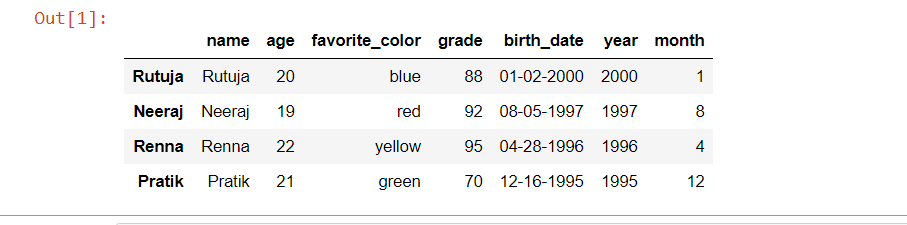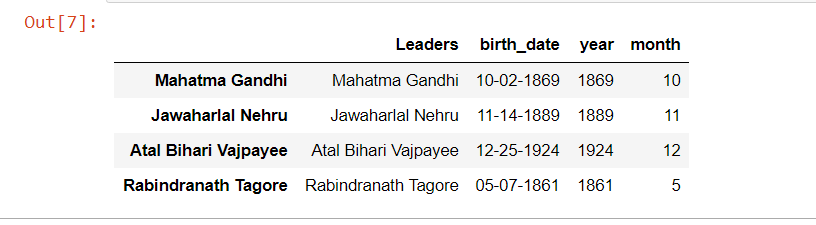# Get month and Year from Date in Pandas – Python

Pandas is one of the most powerful library in Python which is used for high performance and speed of calculation. It is basically an open-source BSD-licensed Python library. Commonly it is used for exploratory data analysis, machine learning, data visualization in data science, and many more. It has very dynamic and easy to understand syntax which makes users jobs easier and is a boost for developers’ innovations (as pandas is a open-source library).

Let us now start with installing pandas. Following are the commands for installing pandas on Linux, windows or mac directly use:

```pip install pandas
```

For installing pandas on anaconda environment use:

```conda install pandas
```

Lets now load pandas library in our programming environment.

```import pandas as pd
```

Coming to accessing month and date in pandas, this is the part of exploratory data analysis. Suppose we want to access only the month, day, or year from date, we generally use pandas.

Method 1: Use DatetimeIndex.month attribute to find the month and use DatetimeIndex.year attribute to find the year present in the Date.

```df['year'] = pd.DatetimeIndex(df['Date Attribute']).year
df['month'] = pd.DatetimeIndex(df['Date Attribute']).month
```

Here ‘df’ is the object of the dataframe of pandas, pandas is callable as ‘pd’ (as imported), ‘DatatimeIndex()’ is a function in pandas which is used to refer to the date attribute of your dataset, ‘Date Attribute’ is the date column in your data-set (It can be anything ans varies from one data-set to other), ‘year’ and ‘month’ are the attributes for referring to the year and month respectively.
Let’s now look at an example:

Code :

## Python3

 `# import pamdas library ` `import` `pandas as pd ` ` `  ` `  `# dictionary of string  key and list value ` `raw_data ``=` `{``'name'``: [``'Rutuja'``, ``'Neeraj'``, ` `                     ``'Renna'``, ``'Pratik'``], ` `            ``'age'``: [``20``, ``19``, ``22``, ``21``], ` `            ``'favorite_color'``: [``'blue'``, ``'red'``,  ` `                               ``'yellow'``, ``"green"``], ` `            ``'grade'``: [``88``, ``92``, ``95``, ``70``], ` `            ``'birth_date'``: [``'01-02-2000'``, ``'08-05-1997'``, ` `                           ``'04-28-1996'``, ``'12-16-1995'``]} ` ` `  `# create a dataframe object ` `df ``=` `pd.DataFrame(raw_data,  ` `                  ``index ``=` `[``'Rutuja'``, ``'Neeraj'``,  ` `                           ``'Renna'``, ``'Pratik'``]) ` ` `  `# get year from the corresponding  ` `# birth_date column value ` `df[``'year'``] ``=` `pd.DatetimeIndex(df[``'birth_date'``]).year ` ` `  `# get month from the corresponding  ` `# birth_date column value ` `df[``'month'``] ``=` `pd.DatetimeIndex(df[``'birth_date'``]).month ` ` `  `# Show the dataframe ` `# by default 5 rows from top  ` `df.head() `

Output:So in the output it is clearly seen that the last two columns of the data-set are appended and we have separately stored the month and date using pandas.

Method 2:  Use datetime.month attribute to find the month and use datetime.year attribute to find the year present in the Date .

```df['year'] = df['Date Attribute'].dt.year
df['month'] = df['Date Attribute'].dt.month
```

Here ‘df’ is the object of the dataframe of pandas, pandas is callable as ‘pd’ (as imported), datetime is callable as ‘dt’ (as imported). ‘Date Attribute’ is the date column in your data-set (It can be anything ans varies from one data-set to other), ‘year’ and ‘month’ are the attributes for referring to the year and month respectively.
Let’s now look at example:

Code:

## Python3

 `# import required library ` `import` `pandas as pd ` `import` `datetime as dt ` ` `  `# dictionary of string as key  ` `# and list as a value ` `raw_data ``=` `{``'Leaders'``: [``'Mahatma Gandhi'``, ``'Jawaharlal Nehru'``, ` `                        ``'Atal Bihari Vajpayee'``, ``'Rabindranath Tagore'``], ` `            ``'birth_date'``: [``'10-02-1869'``, ``'11-14-1889'``, ` `                           ``'12-25-1924'``, ``'05-07-1861'``]} ` ` `  `# create a dataframe object ` `df ``=` `pd.DataFrame(raw_data,  ` `                  ``index ``=` `[``'Mahatma Gandhi'``, ``'Jawaharlal Nehru'``, ` `                           ``'Atal Bihari Vajpayee'``, ` `                           ``'Rabindranath Tagore'``]) ` ` `  `# get a year from corresponding ` `# birth_date column value ` `df[``'year'``] ``=` `df[``'birth_date'``].dt.year ` ` `  `# get a month from corresponding ` `# birth_date column value ` `df[``'month'``] ``=` `df[``'birth_date'``].dt.month ` ` `  `# show the dataframe ` `# by default first 5 rows  ` `# from top ` `df.head() `

Output:So in the output, it is clearly seen that the last two columns of the data-set are appended and we have separately stored the month and date using pandas.

Attention geek! Strengthen your foundations with the Python Programming Foundation Course and learn the basics.

To begin with, your interview preparations Enhance your Data Structures concepts with the Python DS Course.

My Personal Notes arrow_drop_upEnthusiast Technical Content Writer

If you like GeeksforGeeks and would like to contribute, you can also write an article using contribute.geeksforgeeks.org or mail your article to contribute@geeksforgeeks.org. See your article appearing on the GeeksforGeeks main page and help other Geeks.

Please Improve this article if you find anything incorrect by clicking on the "Improve Article" button below.

Article Tags :

Be the First to upvote.

Please write to us at contribute@geeksforgeeks.org to report any issue with the above content.